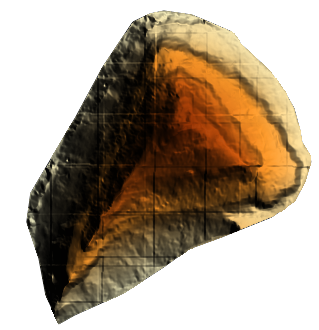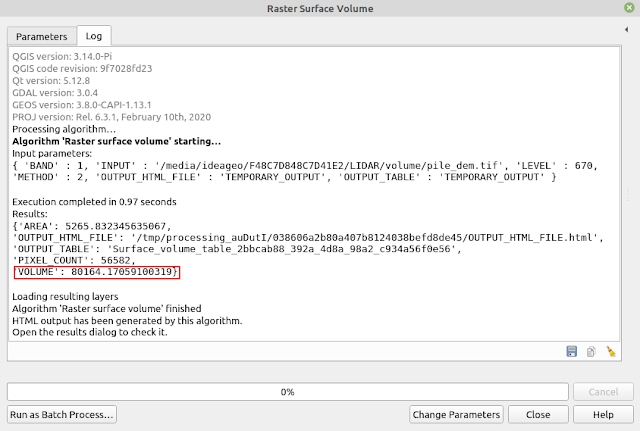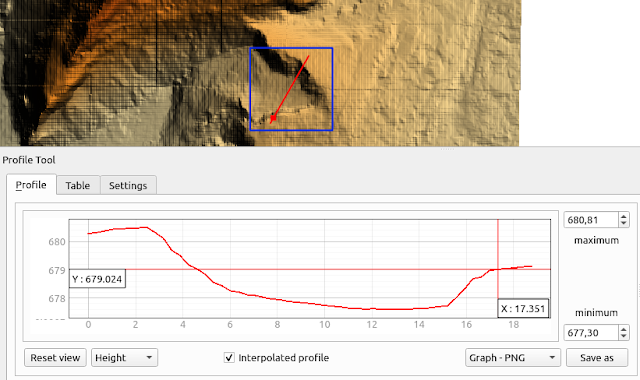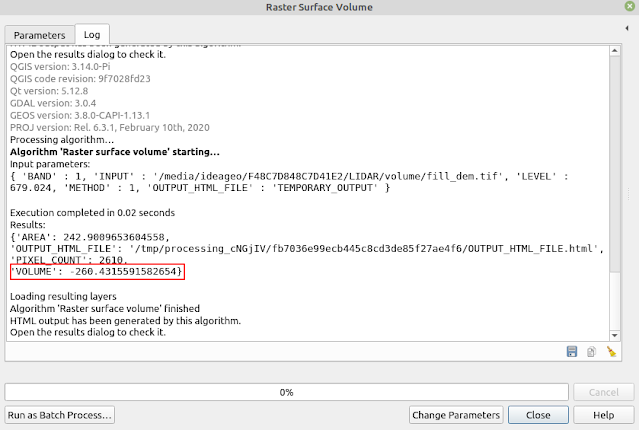# How to Calculate Raster Surface Volume in QGIS

Calculating volume is one of main tasks when working with terrain or topographic data. In other posts I already discussed some topics related to terrain data processing like creating DEM from point dataset, creating profile and visualize DEM data in 3D. In this tutorial I would like to explain how to calculate volume in QGIS. I will discuss some topics such as raster volume algorithm calculation, how to calculate raster volume in QGIS and cut/fill volume.

### Raster Volume Calculation Algorithm

Calculating volume using raster data has a simple algorithm which is area of a pixel(p) multiply with the value(h) of respective pixel that contains the height information. While a raster which the volume will be calculated comprises of many pixel, then the total volume is summation of each pixel area multiply with respective pixel value as shown in the following formula $V={\sum_{i=1}^n p_i*h_i}$ Area of a pixel depends on pixel dimension which is called pixel resolution. A raster with a high resolution like Digital Elevation Model(DEM) from drone survey with 0.1 m resolution compare with DEM SRTM with 30 m resolution will give very different result,  because a pixel of DEM from drone survey represents an area 0.1 m x 0.1 m, while a pixel of SRTM DEM represents area 30 m x 30 m. Which one is more accurate? Of course DEM from drone survey will give more accurate result compare to the SRTM. That's why in a project that is required volume calculation, a raster with higher resolution is preferred.

Figure 1 shows 2D profile of an object which related to different raster resolution. In the figure, can be seen that a raster with higher resolution represents the actual topographic surface closely compare to the other one with lower resolution.Figure 1. Raster resolution and volume accuracy

### Volume Base Level

Next, we will discuss about base level, what is it actually? Base level is a reference level from where the height will be calculated. In the previous formula we can see that a volume of a pixel is the product of pixel area with the pixel value. The pixel value contains the height information. Next question is, from where the height is measured? On the other way, where is zero value of height that a pixel is referenced from?

In technical term the zero point of height value is called vertical datum. There are several vertical datums can be used as height reference such as Mean Sea Level (MSL), Geoid, Ellipsoid model and local vertical datum. Then what it has to do with volume calculation? In a real application, it is really rare that a volume is calculated from vertical datum because the zero height is not presents in a DEM data, and usually we only calculate a volume of object from it's foot.

To get a volume of specific object area or object in a raster of DEM data, we need to determine a base level to where the volume is referenced from. Then how this base level is applied in the volume calculation? After a base level is specified, it will be used to get the height of a pixel from the base level. The pixel value will be subtracted with the base level value. If a base level has symbol $b_0$ then the formula for raster volume calculation will be $V={\sum_{i=1}^n p_i*(h_i-b_0)}$Figure 2. Volume and base level reference

Figure 2 shows relation among Mean Sea Level (MSL) as vertical datum, base level and volume. If a volume that to be calculated without a base level is determined then the volume is referenced from the datum. When a base level is determined then the volume can be calculated from the base level both above or below base level.

### How to Calculate Raster Surface Volume in QGIS

After knowing the algorithm how to calculate a surface volume, next let's compute it. In this tutorial we will calculate the volume of a pile as shown in figure 3 using GIS open source software QGIS. For this tutorial I'm using QGIS 3.14 Pi.Figure 3. DEM data for volume calculation

To make sure only the volume of the pile will be calculated, it's recommended to clip only the pile DEM data. This can be achieved with two steps.

1. Digitize a polygon area for the pile.
2. Clip the DEM based on the polygon using Clip Raster by Mask Layer tool, that can be found in Raster >> Extraction.

After finishing those two steps, the result of clipped raster data can be seen as in figure 4.Figure 4. Clipped DEM

To calcualte raster surface volume in QGIS can be used Raster surface volume tool with the following steps.

1. Open Processing Toolbox, then select Raster analysis and choose Raster surface volume as in figure 5.Figure 5. Raster surface volume tool

2. The Raster surface volume window will appear as in figure 6. In Input layer parameter, select a DEM to be calculated, for my case pile_dem. Then select Base level value. I gave 670, because the minimum raster value (height value) of the DEM data is 670 m. Next in Method parameter, select a method to be used. There area several methods available such as: Count Only Above Base Level (A), Count Only Below Base Level (B), Subtract Volumes Below Base Level (A-B) and Add Volumes Below Base Level (A+B). Since I want to calculate the volume of the pile from the bottom, then I chose Count Only Above Base Level. Lastly for output parameter it can be saved to a file or in temporary file.Figure 6. Raster surface volume window

3. Click Run button. The process will running. When it's done, the result can be seen in the Log tab as in Figure 7.FIgure 7. Volume result

In the result can be seen some records like area of raster surface, pixel count and volume. What is area and volume unit? It follows the unit of DEM data. If it is in meter both for pixel size and height, then the area is in square meter and volume in cubic meter. Therefore it's really important to check the coordinate system of the data first, before calculating the volume. If the coordinate system has an unprojected coordinate system like Geographic coordinate system which has degree unit, it's better to reproject it into a projected coordinate system like Universal Transverse Mercator(UTM).

### How to Calculate Cut and Fill Volume

In this section we will discuss about cut and fill volume calculation. What is cut or fill volume? Figure 8 shows the difference between cut and fill volume. Cut volume is a volume of a mass object. On the other hand, fill volume is a volume of empty object. In the previous step we already calculated a pile volume, which is a cut volume. What about fill volume, how to calculate it?Figure 8. Cut and Fill Volume

In this tutorial we will calculate fill volume of a small polygon area  from the previous DEM data as shown in figure 9. The figure also shows the 2D profile along the red line. From the profile we will calculate fill volume below 679.024 m base level.Figure 9. Fill volume and profile line

To calculate the fill volume below a base level can be done with following steps:

1. Clip area of interest using a polygon where a fill volume to be calculated.
2. Open the Raster Surface Volume tool. Select the input layer and determined the base level. In this case I want to calculate the fill volume below 679.024 m. In the Method parameter choose Count Only Below Base Level.
3. Click the Run button. The result can be seen in the Log tab as in figure 10.Figure 10. Fill volume result

The fill volume is 260.432 cubic meter. The minus sign can be indicated as fill volume. This is because the height of DEM ($h_i$) is below the base level ($b_0$).

That's all tutorial how to calculate raster surface volume in QGIS using Raster surface volume tool. In this tutorial we had learnt the algorithm how to compute volume in raster data, base level, calculate fill and cut volume. Thanks for reading!